### NCERT Solutions For Class 9 Math Chapter – 6 Exercise – 6.2

NCERT Solutions For Class 9 Math Chapter – 6 Exercise – 6.2

Q1. In Fig 6.28 Find the value of x and y and then show that AB,CD.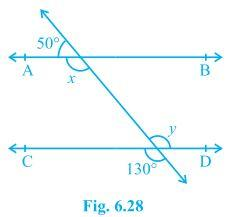Solution:-

X + 500 = 1800

X = 1300

We also know that vertically opposite angles are equal.

Y = 1300

In two parallel lines , the  alternate interior angle are equal In this ,

X = y = 1300

This proves that alternate interior angles are equal. In this ,

X = y = 1300

This proves that alternate interior angles are equal and so AB , CD

Q2. In Fig 6.29 If AB   CD , CE EF and y : z = 3:7  find x.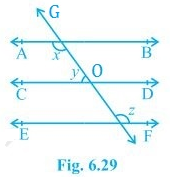Solution:-

X + y = 1800 (I)

0 = z (Since they are corresponding angles)

Y + 0 = 1800 (Since they are a linear pair)

Y + z = 1800

Y = 3w  z = 7w (As y:z = 3:7)

3w + 7w =  1800

10w = 1800

W = 180

Y = 3 x 180 = 540

Z = 7 x 180 = 1260

X + y = 1800 (I)

X + 540 = 1800

X = 1260

Q3. In Fig 6.30 If AB  CD, EF bisect CD and GED = 1260 Find AGE , GEF and FGE.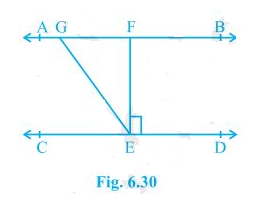Solution:-

GED = AGE = 1260 (As they are alternate interior angels)

Also,

GED = GEF + FED

As EF  bisects CD, FED = 900

GED = GEF + 900

GEF = 1260 – 900 = 360

FGE + GED = 1800 (Transversal)

GED = 1260

FGE = 540

AGE = 1260

GEF = 360 AND FGE = 540

Q4. In Fig 6.31 If PQ ST , PQR = 1100 and RST = 1300 Find QRS.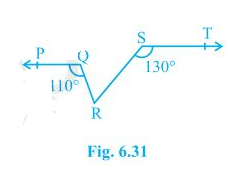Solution:-

First, construct a line XY parallel to PQ.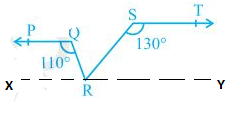We know that the angles on the same side of transversal is equal to 180°.

So, PQR+QRX = 180°

Or,QRX = 180°-110°

∴ QRX = 70°

Similarly,

RST +SRY = 180°

Or, SRY = 180°- 130°

∴ SRY = 50°

Now, for the linear pairs on the line XY-

QRX+QRS+SRY = 180°

Putting their respective values, we get,

QRS = 180° – 70° – 50°

Hence, QRS = 60°

1. In Fig. 6.32, if AB CD, APQ = 50° and PRD = 127°, find x and y.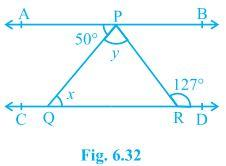Solution:

From the diagram,

APQ = PQR (Alternate interior angles)

Now, putting the value of APQ = 50° and PQR = x we get,

x = 50°

Also,

APR = PRD (Alternate interior angles)

Or, APR = 127° (As it is given that PRD = 127°)

We know that

APR = APQ+QPR

Now, putting values of QPR = y and APR = 127° we get,

127° = 50°+ y

Or, y = 77°

Thus, the values of x and y are calculated as:

x = 50° and y = 77°

1. In Fig. 6.33, PQ and RS are two mirrors placed parallel to each other. An incident ray AB strikes the mirror PQ at B, the reflected ray moves along the path BC and strikes the mirror RS at C and again reflects back along CD. Prove that AB CD.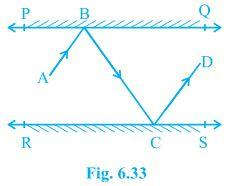Solution:

First, draw two lines BE and CF such that BE ⊥ PQ and CF ⊥ RS.

Now, since PQ RS,

So, BE CF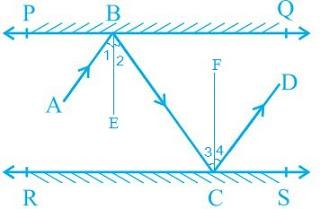We know that,

Angle of incidence = Angle of reflection (By the law of reflection)

So,

1 = 2 and

3 = 4

We also know that alternate interior angles are equal. Here, BE ⊥ CF and the transversal line BC cuts them at B and C

So, 2 = 3 (As they are alternate interior angles)

Now, 1 +2 = 3 +4

Or, ABC = DCB

So, AB CD alternate interior angles are equal)